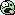# Rest energy of the electron

• Mizies

#### Mizies

Electrons in a particle accelerator reach a speed of 0.999c relative to the laboratory. Is the rest energy of the electron equal to zero?

The kinetic energy of an electron (or anything else, for that matter) at rest is zero.

The total energy of the electron, calculated from ##E=\gamma{m_0}c^2## or ##E^2=(m_0c^2)^2+(pc)^2"##, is .511 511 MeV when the electron is at rest and greater when it is moving and the kinetic energy is non-zero.

[Edit - left the decimal point out the first time around]

Last edited:
Is the rest energy of the electron equal to zero?

The rest energy of an electron is 0.511 MeV, regardless of how fast it is moving.

The kinetic energy of an electron (or anything else, for that matter) at rest is zero.

The total energy of the electron, calculated from ##E=\gamma{m_0}c^2## or ##E^2=(m_0c^2)^2+(pc)^2"##, is 511 MeV when the electron is at rest and greater when it is moving and the kinetic energy is non-zero.

.511MeV or 511keV bro, 511MeV is some ginormous electron.

•Nugatory
.511MeV or 511keV bro, 511MeV is some ginormous electron.
:)

511MeV is some ginormous electron.

It's overweight even for a muon.thanks..